Next: Brillouin Precursor Up: Wave Propagation in Uniform Previous: Method of Stationary Phase

# Group Velocity

The point of stationary phase, defined by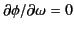, satisfies the condition(918)

where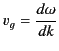(919)

is conventionally termed the group velocity. Thus, the signal seen at position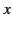and timeis dominated by the frequency range whose group velocity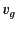is equal to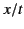. In this respect, the signal incident at the surface of the medium (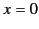) at time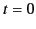can be said to propagate through the medium at the group velocity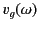.

The simple one-resonance dielectric dispersion relation (871) yields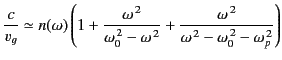(920)

in the limit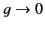, where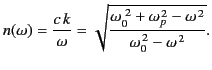(921)

The variation of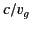, and the refractive index, with frequency is sketched in Figure 12. With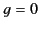, the group velocity is less than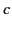for all, except for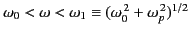, where it is purely imaginary. Note that the refractive index is also complex in this frequency range. The phase velocity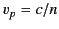is subluminal for, imaginary for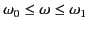, and superluminal for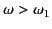.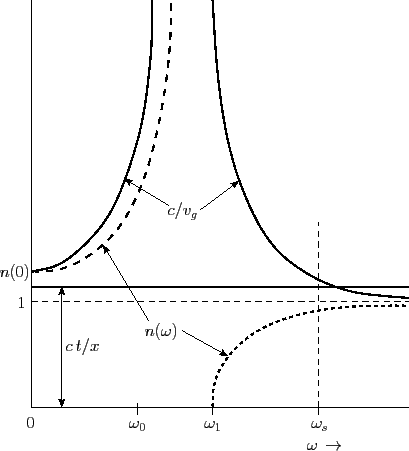The frequency range that contributes to the amplitude at timeis determined graphically by finding the intersection of a horizontal line with ordinate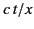with the solid curve in Figure 12. There is no crossing of the two curves for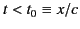. Thus, no signal can arrive before this time. For times immediately after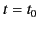, the point of stationary phase is seen to be at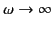. In this large-limit, the point of stationary phase satisfies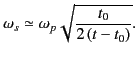(922)

Note that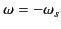is also a point of stationary phase. It is easily demonstrated that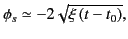(923)

and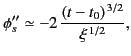(924)

with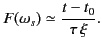(925)

Here,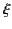is given by Equation (894). The stationary phase approximation (918) yields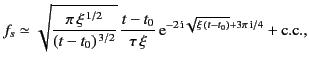(926)

where c.c. denotes the complex conjugate of the preceding term (this contribution comes from the second point of stationary phase located at). The previous expression reduces to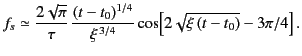(927)

It is readily shown that the previous formula is the same as expression (903) for the Sommerfeld precursor in the large argument limit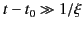. Thus, the method of stationary phase yields an expression for the Sommerfeld precursor that is accurate at all times except those immediately following the first arrival of the signal.Next: Brillouin Precursor Up: Wave Propagation in Uniform Previous: Method of Stationary Phase
Richard Fitzpatrick 2014-06-27